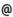## Babes-Bolyai University of Cluj-Napoca Faculty of Mathematics and Computer Science Study Cycle: Graduate SUBJECT

 Code Subject MME0003 Mathematical Modelling
 Section Semester Hours: C+S+L Category Type Applied Mathematics 4 2+2+0 speciality compulsory
 Teaching Staff in Charge
 Lect. SERBAN Marcel Adrian, Ph.D.,  mserbanmath.ubbcluj.roAssoc.Prof. BUICA Adriana, Ph.D.,  abuicamath.ubbcluj.ro
 Aims This course is an introduction in the mathematical modeling. There are presented basic notions of mathematical modeling process in order to analyse, study and construct mathematical models.
 Content I. Mathematical modelling process 1. Introduction: Important steps in the mathematical history 2. Relation between mathematics and its applications 3. Mathematical modelling process 4. Simple mathematical models: a) Transports problem; b) Fibonacci's problem; c) Bank interest; d) Marketing expenses optimation II. Dynamical systems 1. The notion of dynamical system 2. Dynamical systems generated by difference equations 3. Stationary steady, stability III. Discrete mathematical models 1. Linear difference equations with constant coeficients 2. Linear systems of difference equations with constant coeficients 3. Discrete mathematical models. The logistic difference equation, discrete multispecies models IV. Populations dynamics 1. Continuous dynamical systems 2. Autonomuous systems, stability of equilibrium solutions 3. Mathematical models for a population dynamics (Malthus, Verhulst, harvesting models) 4. Multispecies models (prey-predator, competition, symbiosis) V. Mathematical models in epidemiology 1. Epidemiological models of SIR and SIRS type 2. Models in study of spreading an infectious desease.
 References 1. RUS, IOAN A. - IANCU, CRACIUN: Modelare matematica, Editura Transilvania, Cluj-Napoca, 2000 2. IANCU, CRACIUN: Modelare matematica. Teme speciale. Ed. Casa Cartii de Stiinta, Cluj-Napoca, 2002 3. MURRAY,J.D.: Mathematical biology, Springer-Verlag, Berlin,1989. 4. FOWLER, A.C.: Mathematical models in applied sciences, Cambridge University Press, 1989. 5. AGARWAL, R.P., Difference equations and inequalities, 2nd Edition, Theory, Methods and Applications, Marcel Dekker Inc. 2000 6. Arrowsmith, Dynamical systems, Differential equations, maps and chaotic behaviour, Chapmann and Hall, 1992
 Assessment During the semester one test with 20% from final degree and final exam with 80% from final degree.
 Links: Syllabus for all subjects Romanian version for this subject Rtf format for this subject